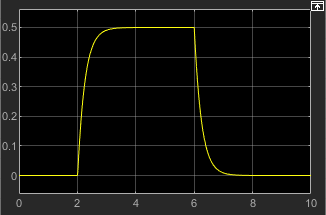## Creating and Simulating a Simple Model

### Building a Simscape Diagram

In this example, you are going to model a simple mechanical system and observe its behavior under various conditions. This tutorial illustrates the essential steps to building a physical model and makes you familiar with using the basic Simscape™ blocks.

Note

For time-saving techniques and advanced ways of analyzing simulation data, see the Essential Steps for Constructing a Physical Model tutorial.

The following schematic represents a simple model of a car suspension. It consists of a spring and damper connected to a body (represented as a mass), which is agitated by a force. You can vary the model parameters, such as the stiffness of the spring, the mass of the body, or the force profile, and view the resulting changes to the velocity and position of the body.To create an equivalent Simscape diagram, follow these steps:

1. Open the Simulink® Library Browser, as described in Simscape Block Libraries.

2. Create a new Simulink model using the Blank Model template. The software creates an empty model in memory and displays it in a new model editor window.

Note

Alternatively, you can type `ssc_new` at the MATLAB® Command prompt, to create a new model prepopulated with certain required and commonly used blocks. For more information, see Creating a New Simscape Model.

3. By default, Simulink Editor hides the automatic block names in model diagrams. To display hidden block names for training purposes, clear the Hide Automatic Block Names check box. For more information, see Configure Model Element Names and Labels.

4. Open the Simscape > Foundation Library > Mechanical > Translational Elements library.

5. Drag the Mass, Translational Spring, Translational Damper, and two Mechanical Translational Reference blocks into the model window.

6. Orient the blocks as shown in the following illustration. To rotate a block, select it and press Ctrl+R.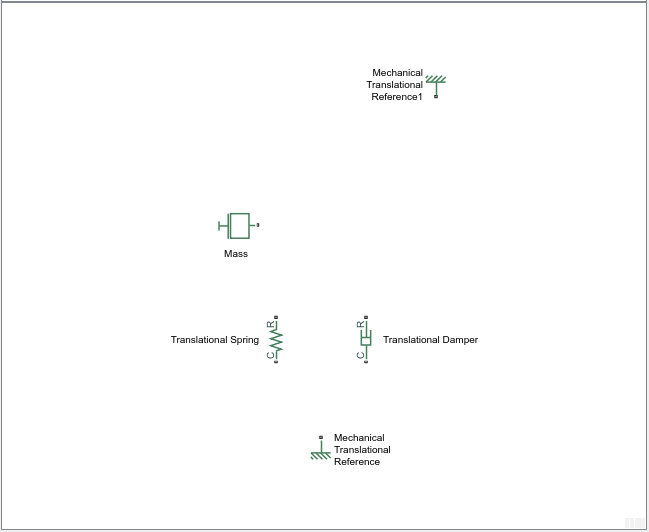7. Connect the Translational Spring, Translational Damper, and Mass blocks to one of the Mechanical Translational Reference blocks as shown in the next illustration.8. To add the representation of the force acting on the mass, open the Simscape > Foundation Library > Mechanical > Mechanical Sources library and add the Ideal Force Source block to your diagram.

To reflect the correct direction of the force shown in the original schematic, flip the block orientation. With the Ideal Force Source block selected, on the Format tab at the top of the model window, under Arrange, click Flip up-down. Connect the block's port C (for “case”) to the second Mechanical Translational Reference block, and its port R (for “rod”) to the Mass block, as shown below.9. Add the sensor to measure speed and position of the mass. Place the Ideal Translational Motion Sensor block from the Mechanical Sensors library into your diagram and connect it as shown below.10. Now you need to add the sources and scopes. They are found in the Simulink libraries. Open the Simulink > Sources library and copy the Signal Editor block into the model. Then open the Simulink > Sinks library and copy two Scope blocks. Rename one of the Scope blocks to `Velocity` and the other to `Position`.11. Every time you connect a Simulink source or scope to a Simscape diagram, you have to use an appropriate converter block, to convert Simulink signals into physical signals and vice versa. Open the Simscape > Utilities library and copy a Simulink-PS Converter block and two PS-Simulink Converter blocks into the model. Connect the blocks as shown below.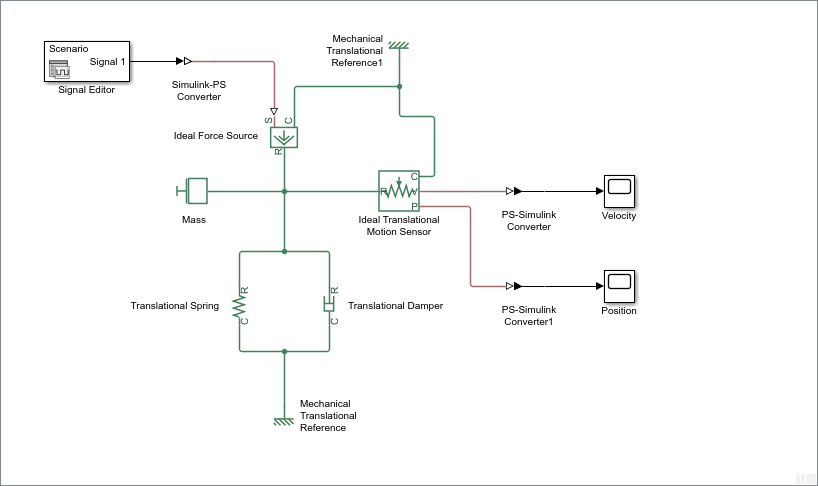12. Each topologically distinct physical network in a diagram requires exactly one Solver Configuration block, found in the Simscape > Utilities library. Copy this block into your model and connect it to the circuit by creating a branching point and connecting it to the only port of the Solver Configuration block. Your diagram now should look like this.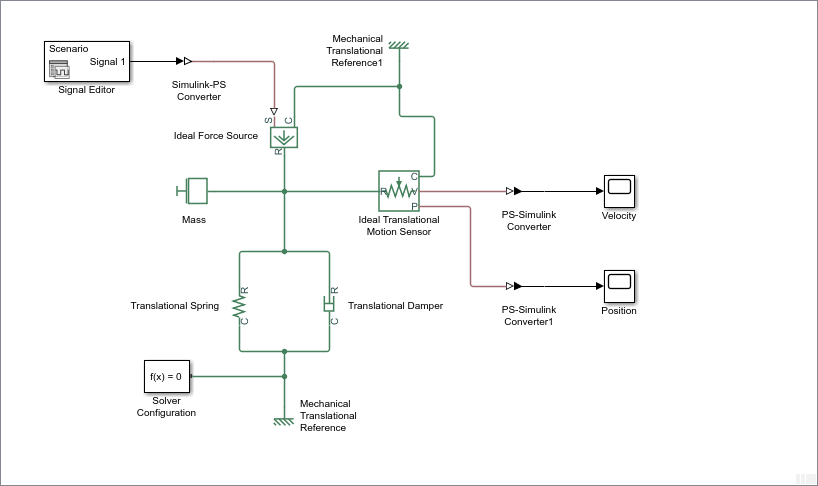13. Your block diagram is now complete. Save it as `mech_simple`.

### Modifying Initial Settings

After you have put together a block diagram of your model, as described in the previous section, you need to select a solver and provide the correct values for configuration parameters.

To prepare for simulating the model, follow these steps:

1. Select a Simulink solver. In the model window, open the Modeling tab and click Model Settings. The Configuration Parameters dialog box opens, showing the Solver pane.

Under Solver selection, set Solver to `ode23t (mod.stiff/Trapezoidal)`.

Expand Solver details and set Max step size to `0.2`.

Also note that Simulation time is specified to be between 0 and 10 seconds. You can adjust this setting later, if needed.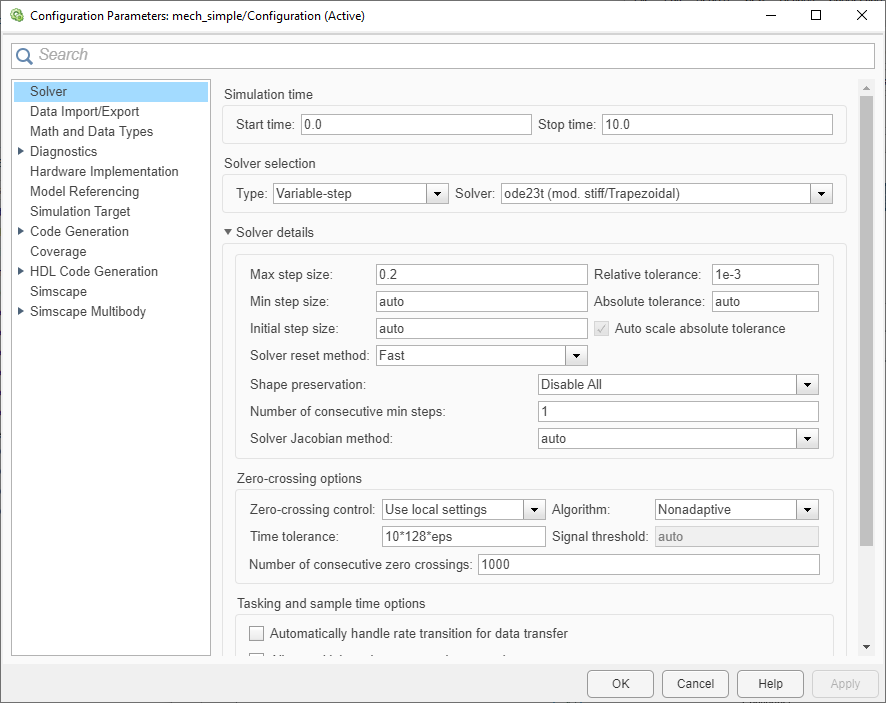Click to close the Configuration Parameters dialog box.

2. Save the model.

### Running the Simulation

After you've put together a block diagram and specified the initial settings for your model, you can run the simulation.

1. The input signal for the force is provided by the Signal Editor block. Edit the signal properties as shown in the illustration below. The signal profile starts with a value of 0, then at 4 seconds there is a step change to 1, and then it changes back to 0 at 6 seconds.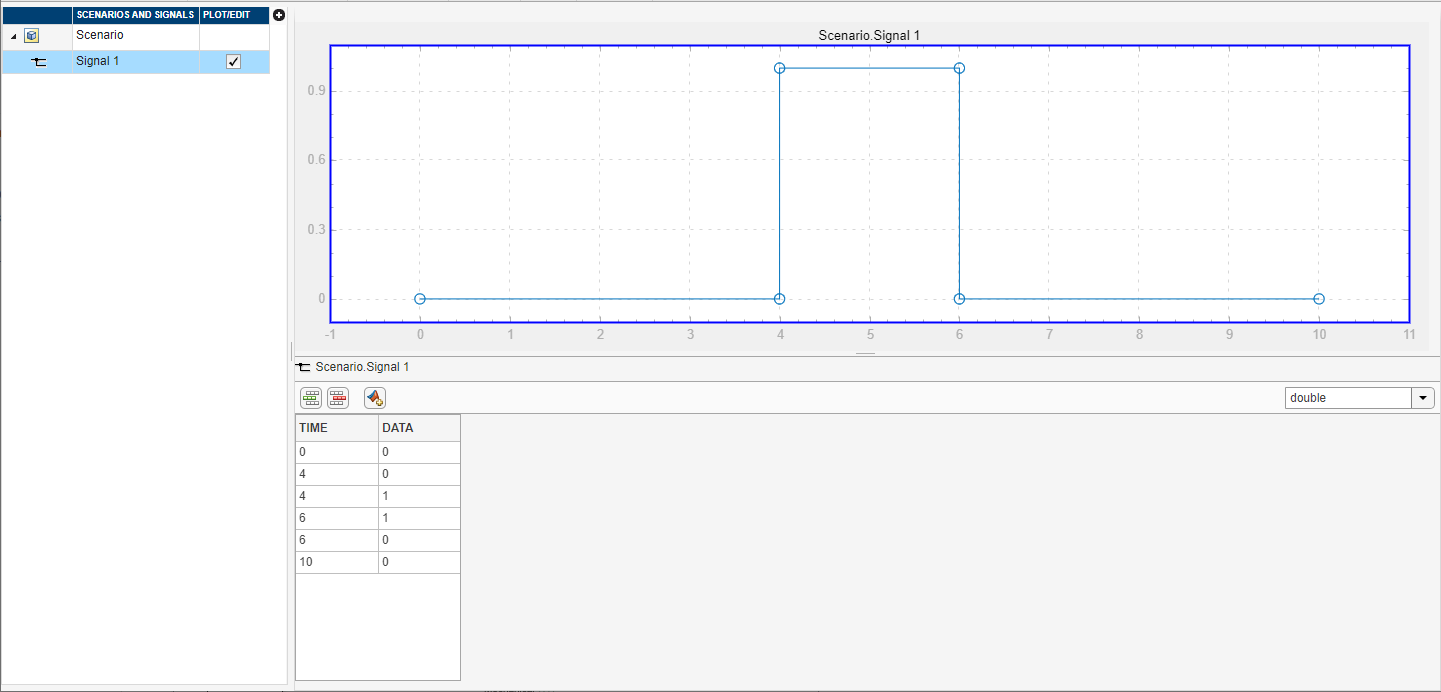The Velocity scope outputs the mass velocity, and the Position scope outputs the mass displacement as a function of time. Double-click both scopes to open them.

2. Click. The Simscape solver evaluates the model, calculates the initial conditions, and runs the simulation. For a detailed description of this process, see How Simscape Simulation Works. Completion of this step may take a few seconds. The message in the bottom-left corner of the model window provides the status update.

3. Once the simulation starts running, the Velocity and Position scope windows display the simulation results, as shown in the next illustration.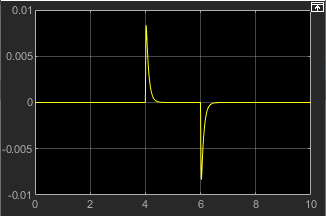In the beginning, the mass is at rest. Then at 4 seconds, as the input signal changes abruptly, the mass velocity spikes in the positive direction and gradually returns to zero. The mass position at the same time changes more gradually, on account of inertia and damping, and stays at the new value as long as the force is acting upon it. At 6 seconds, when the input signal changes back to zero, the velocity gets a mirror spike, and the mass gradually returns to its initial position.

You can now adjust various inputs and block parameters and see their effect on the mass velocity and displacement.

After running the initial simulation, you can experiment with adjusting various inputs and block parameters.

#### Changing the Force Profile

This example shows how a change in the input signal affects the force profile, and therefore the mass displacement.

1. Double-click the Signal Editor block to open it.

2. Change the signal profile by moving its first vertical segment from 4 to 2 seconds, as shown below. Close the block dialog.3. Run the simulation. The simulation results are shown in the following illustration.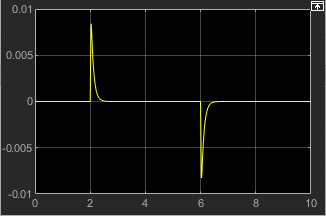#### Changing the Model Parameters

In our model, the force acts on a mass against a translational spring and damper, connected in parallel. This example shows how changes in the spring stiffness and damper viscosity affect the mass displacement.

1. Double-click the Translational Spring block. Set its Spring rate to `2000 N/m`.

2. Run the simulation. The increase in spring stiffness results in smaller amplitude of mass displacement, as shown in the following illustration.3. Next, double-click the Translational Damper block. Set its Damping coefficient to ```500 N/(m/s)```.

4. Run the simulation. Because of the increase in viscosity, the mass is slower both in reaching its maximum displacement and in returning to the initial position, as shown in the following illustration.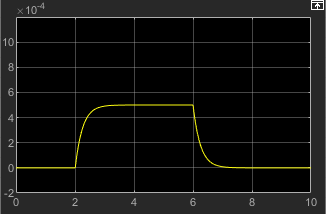#### Changing the Mass Position Output Units

In our model, we have used the PS-Simulink Converter block in its default parameter configuration. Therefore, the `Position` scope outputs the mass displacement in the default length units, that is, in meters. This example shows how to change the output units for the mass displacement to millimeters.

1. Double-click the PS-Simulink Converter1 block. Type `mm` in the Output signal unit combo box and click .

2. Run the simulation. In the `Position` scope window, clickto autoscale the scope Y-axis. The mass displacement is now output in millimeters, as shown in the following illustration.